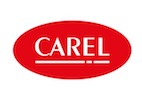# BTL Listing of Tested Products

### Carel Industries S.p.A.

www.carel.com#### BACnet Building Controller (B-BC)

 Product Model Version PICS BTL Listing Certificate c.pCO Family P+5 XX1 X2 X3 X4 X5 X6X7X8X9 where XX1 is 00, 04, 0N, K0, HV, W0, A0, PX, DN, AD, BB, DK or EP X2 is S X3 is B, E, F, M, N, S, T. U or V X4 is A, B, C, D, E, F, G, H, Z, 7, 8 or 9 X5 is 0, 1, 2, 3, 4, 5, 6, A, B, C, D, E, F or Z X6 is 0, 1, 2, 3, 4, 5, 6, 7 or 8 X7 is 0 or E X8 is X, S, M, L, C, Z or J X9 is 0, 1, 2 or K P+D XX1 X2 X3 X4 X5 X6X7X8X9, P+P XX1 X2 X3 X4 X5 X6X7X8X9, P+R XX1 X2 X3 X4 X5 X6X7X8X9 where XX1 is 00, 0N, BB, DK, SV, W0, A0, 0R, 0B, HV or NM X2 is 0 X3 is U, N, B or F X4 is C, B, E or H X5 is 0, 1, A, B, C, D, E, F, G or H X6 is 0 or D X7 is 0, E, B, L or F X8 is F or S X9 is 0, 1, 2 or K 4.8.001c.pCOOEM P+1 XX1 X2 X3 X4 X5 X6X7X8X9 where XX1 is 00 or 0N X2 is S X3 is E, F, N, S, T, U or W X4 is 0 or C X5 is 0, 1, 2, A or B X6 is 0 or D X7 is 0 X8 is S, M or N X9 is 0, 1, 2 or K 4.8.001uPC3 UP3 X1 XX2 X3 X4 X5 X6X7X8X9 where X1 is A, B, C, S, U, V, W, X, Y or Z XX2 is 00, 0B, 02, 0D, AE or DN X3 is 0, 1, 2, 3, 4, 5, A, B, G, H or T X4 is 0, 1, 2, A, B or C X5 is 0, 1, 2, 3, 4, 5, 6, 7, 8, 9, A, R, H, Y or Z X6 is 0, S or T X7 is 2, 3, 4, A, B, C, D or E X8 is X, S, M or L X9 is 0, 1, 2 or K 4.8.001#### BACnet Advanced Application Controller (B-AAC)

 Product Model Version PICS BTL Listing Certificate c.pCO Family P+5 XX1 X2 X3 X4 X5 X6X7X8X9 where XX1 is 00, 04, 0N, K0, HV, W0, A0, PX, DN, AD, BB, DK or EP X2 is S X3 is B, E, F, M, N, S, T. U or V X4 is A, B, C, D, E, F, G, H, Z, 7, 8 or 9 X5 is 0, 1, 2, 3, 4, 5, 6, A, B, C, D, E, F or Z X6 is 0, 1, 2, 3, 4, 5, 6, 7 or 8 X7 is 0 or E X8 is X, S, M, L, C, Z or J X9 is 0, 1, 2 or K P+D XX1 X2 X3 X4 X5 X6X7X8X9, P+P XX1 X2 X3 X4 X5 X6X7X8X9, P+R XX1 X2 X3 X4 X5 X6X7X8X9 where XX1 is 00, 0N, BB, DK, SV, W0, A0, 0R, 0B, HV or NM X2 is 0 X3 is U, N, B or F X4 is C, B, E or H X5 is 0, 1, A, B, C, D, E, F, G or H X6 is 0 or D X7 is 0, E, B, L or F X8 is F or S X9 is 0, 1, 2 or K 4.8.001c.pCOOEM P+1 XX1 X2 X3 X4X5 X6X7X8X9 where XX1 is 00 or 0N X2 is S X3 is E, F, N, S, T, U or W X4 is 0 or C X5 is 0, 1, 2, A or B X6 is 0 or D X7 is 0 X8 is S, M or N X9 is 0, 1, 2 or K 4.8.001uPC3 UP3 X1 XX2 X3 X4 X5 X6X7X8X9 where X1 is A, B, C, S, U, V, W, X, Y or Z XX2 is 00, 0B, 02, 0D, AE or DN X3 is 0, 1, 2, 3, 4, 5, A, B, G, H or T X4 is 0, 1, 2, A, B or C X5 is 0, 1, 2, 3, 4, 5, 6, 7, 8, 9, A, R, H, Y or Z X6 is 0, S or T X7 is 2, 3, 4, A, B, C, D or E X8 is X, S, M or L X9 is 0, 1, 2 or K 4.8.001pCONet Family PCO1 X1 X2 X3 B X4 0 where X1 is 0, A, C, D, E, M, N, R or T X2 is 0, A, B, G, I, N, R, Q, T or W X3 is 0, 1, 2, 3, 4 or P X4 is A or D 2.15.4pCOWeb Family PCO1 X1 X2 X3 W X4 0 where X1 is 0, A, B, C, D, E, G, H, L, M, N, O, R or W X2 is 0, A, B, D, E, J, L, N, O, R, Q, T, V, X or Z X3 is 0, 1, 2, 3, 4 or P X4 is B or D 2.15.4